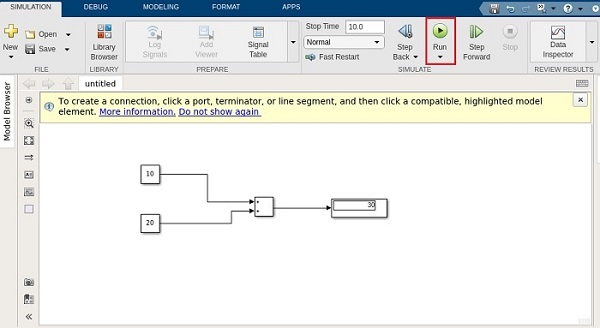# MATLAB Simulink - Lines

#### Artificial Neural Network and Machine Learning using MATLAB

54 Lectures 4 hours

#### Machine Learning for Data Science using MATLAB

Featured

62 Lectures 9 hours

In the previous chapter, we learnt about the different types of blocks which are available with Simulink library. In this chapter, we are going to understand about lines.

Lines are used to connect the blocks with an arrow. Each block will have its own input and output connector. The communication between the blocks will take place with the help of lines.

Let us understand the same with an example. Select a blank model from Simulink page as shown below −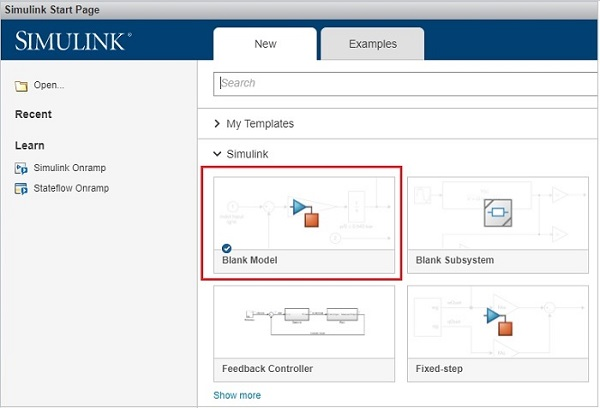It will open a blank model workspace as shown below −Click on Simulink Library browser to drag some blocks in the model workspace.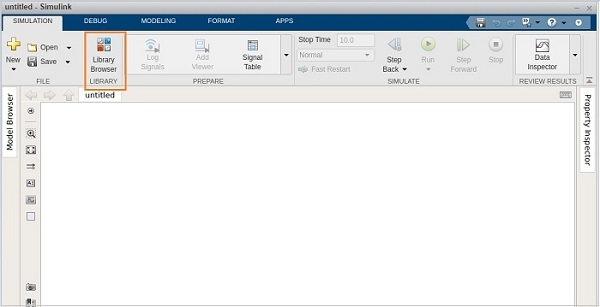Consider that in the model, we want to add two given numbers. So let us pick the Add block, the display block and the constant block.

The constant block has one output connector, the Add block has two input connectors and the display block has one input connector respectively. You can drag the link from one output to another input as shown below.

Here, we have two constants with values 10 and 20. They are connected to the add block with lines. The add block is connected to display with a line.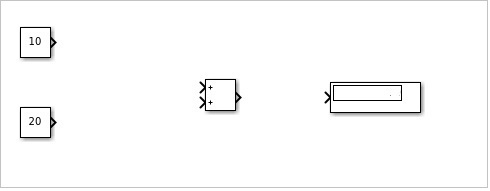When the lines are connected, the display is as follows −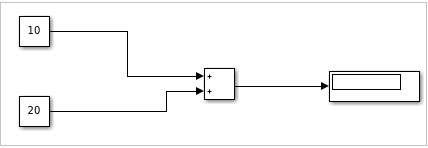Now click on Run to see the result in the display block. It will add 10 + 20 to give the result as 30 in the display block.